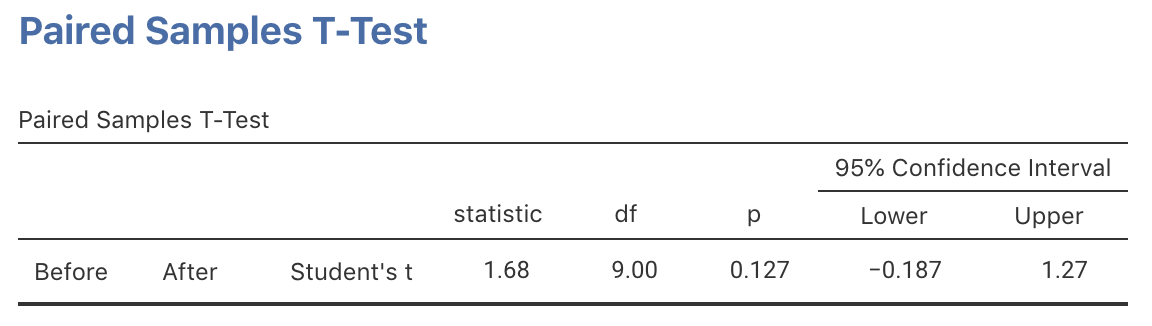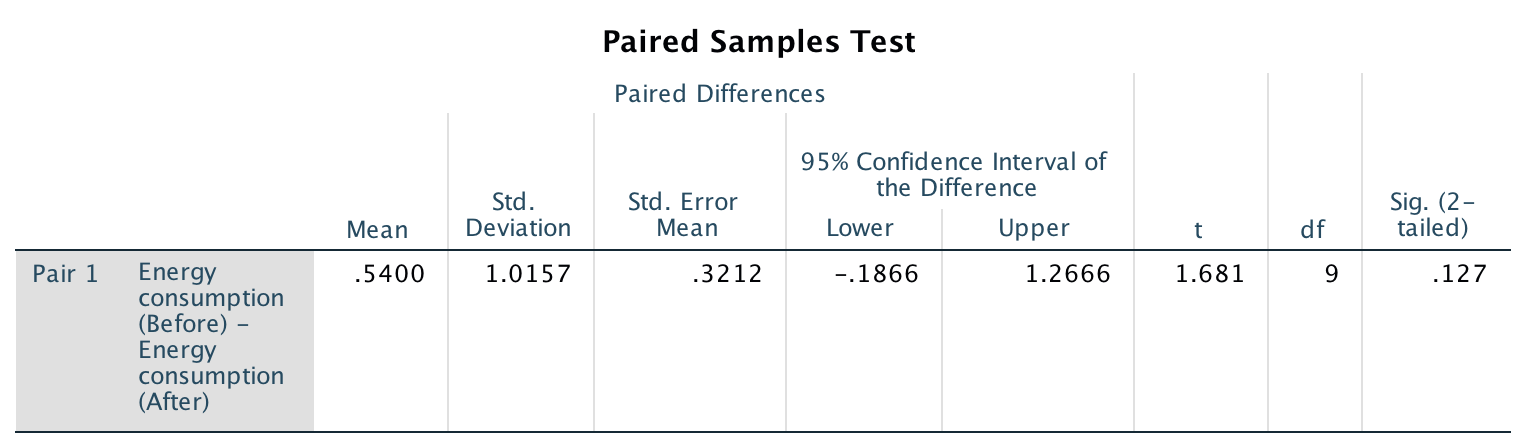## 23.8 Using software: CIs for mean differences

Software (such as jamovi or SPSS) can be used to produce exact 95% CIs, which will may be slightly different than the approximate 95% CI (since the 68–95–99.7 rule is an approximation). The approximate and exact 95% CIs are similar when the sample size is not small; here the sample size is small ($$n=10$$). From the jamovi (Fig. 23.2) or SPSS output (Fig. 23.3):

Based on the sample, a 95% CI is for the population mean energy saving because of the wall cavity insulation is from $$-0.19$$ to $$1.27$$MWh.FIGURE 23.2: The insulation data: jamovi outputFIGURE 23.3: The insulation data: SPSS output

As expected, this 95% CI is slightly different than the CI computed by hand, since the sample size is small. For our purposes, however, using the approximate multiplier of 2 is sufficient when not using software.